# Newton-Cotes quadrature formula

The interpolation quadrature formula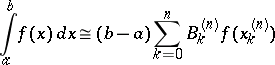for the computation of an integral over a finite interval, with nodes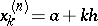,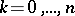, whereis a natural number,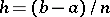, and the number of nodes is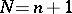. The coefficients are determined by the fact that the quadrature formula is interpolational, that is,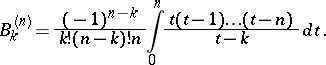For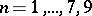all coefficients are positive, for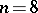and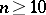there are both positive and negative ones among them. The algebraic degree of accuracy (the numbersuch that the formula is exact for all polynomials of degree at mostand not exact for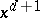) isfor oddandfor even. The simplest special cases of the Newton–Cotes quadrature formula are:,,,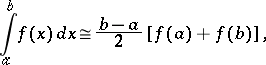the trapezium formula;,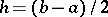,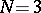,the Simpson formula;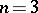,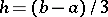,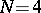,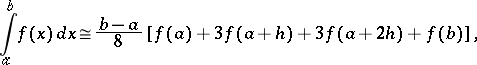the "three-eighths" quadrature formula. For largethe Newton–Cotes formula is seldom used (because of the property of the coefficients formentioned above). One prefers to use for smallthe compound Newton–Cotes quadrature formulas, namely, the trapezium formula and Simpson's formula.

The coefficients of the Newton–Cotes quadrature formula forfrom 1 to 20 are listed in .

The formula first appeared in a letter from I. Newton to G. Leibniz in 1676 (see ) and later in the book  by R. Cotes, where the coefficients of the formula are given forfrom 1 to 10.